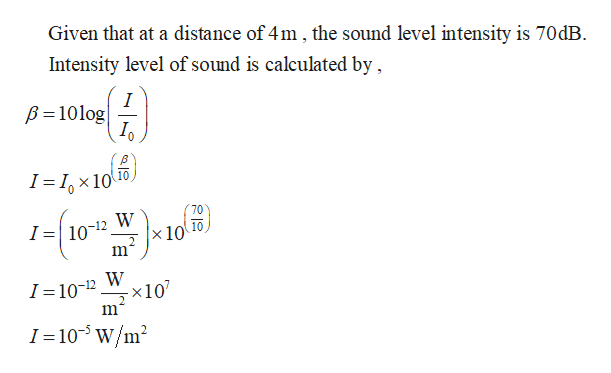# At a distance of 4 m from a certain sound source, the sound level intensity is 70 dB. What is the power being emitted by the sound source? (Assume I0=10−12W/m2.)

Question
1 views

At a distance of 4 m from a certain sound source, the sound level intensity is 70 dB. What is the power being emitted by the sound source? (Assume I0=10−12W/m2.)

check_circle

Step 1help_outlineImage TranscriptioncloseGiven that at a distance of 4m, the sound level intensity is 70dB Intensity level of sound is calculated by I B10log I Ix10 70 I=| 10-12 W x 10 m W -x 10 I =10-12 2 1 105 W/m2 fullscreen

### Want to see the full answer?

See Solution

#### Want to see this answer and more?

Solutions are written by subject experts who are available 24/7. Questions are typically answered within 1 hour.*

See Solution
*Response times may vary by subject and question.
Tagged in

### Physics